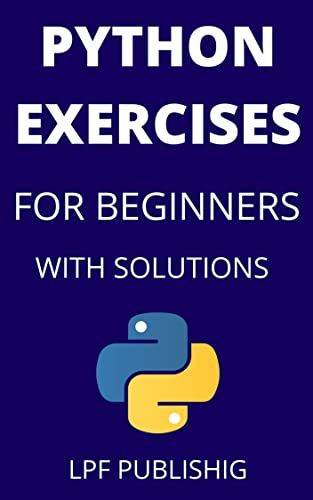Python Coding Exercises For Beginners With SolutionsEnglish | 2021 | ASIN: B09MPM6L2V | 36 pages | PDF,EPUB,AZW3 | 5.99 MB

Are you looking for a hands-on approach to learn Python fast? Or perhaps you have just completed a Python course and are looking for practice questions to test your Python skills.

Then this python exercises book is for you.
This book contains 50+ python exercises with complete solution and output.
Whether you are preparing for a technical interview to get a job as python developer or for scoring in a python exam, this book is all you need.

Most commonly asked interview questions are given in this book with complete solution.
If you want to learn python by doing then this is the book for you. We start with the simplest python programs first and then move on to some complex python programs.
A list of some of the python programs included in this book:

Python Basic Programs

1. Write a python program to print hello world.

2. Write a python program to find area of a triangle

3. Write a python program to swap two variables without using a third variable.

4. Write a python program to generate a random number.

5. Write a python program to check if a number is positive, negative or zero.

6. Write a python program to check if a number is odd or even.

7. Write a python program to find factorial of a number.

8. Write a python program to find lcm.

9. Write a python program to find HCF.

10. Write a python program to find factorial of a number using recursion.

Python Array Programs

1. Write a python program to print the elements of an array.

2. Write a python program to copy all the elements of one array into another array.

3. Write a python program to find the largest element in an array.

4. Write a python program to find the smallest element in an array.

5. Write a python program to print the sum of all elements in an array.

6. Write a python program to print the elements of an array in reverse order.

7. Write a python program to sort the elements of an array in ascending order.

8. Write a python program to sort the elements of an array in descending order.

Python String Programs

1. Write a python program to reverse a string.

2. Write a python program to remove punctuation from a string.

3. Write a python program to convert list to string.

4. Write a python program to convert int to string.

Python list Programs

1. Write a python program to add two lists.

2. Write a python program to append elements in a list.

3. Write a python program to compare two lists in python.

4. Write a python program to remove an element from a list.

Python Searching and Sorting Programs

1. Write a python program to implement linear search.

2. Write a python program to implement binary search.

3. Write a python program to implement bubble sort.

4. Write a python program to implement insertion sort.

5. Write a python program to implement heap sort.

6. Write a python program to implement merge sort.

1. Write a python program to create and display a circular linked list.

2. Write a python program to create a circular linked list of n nodes and count the number of nodes.

3. Write a python program to create a circular linked list of n nodes and display it in reverse order.

4. Write a python program to find the maximum and minimum value node from a circular linked list.

5. Write a python program to remove duplicate elements from a circular linked list.

6. Write a python program to search an element in a circular linked list.

7. Write a python program to sort the elements of a circular linked list.